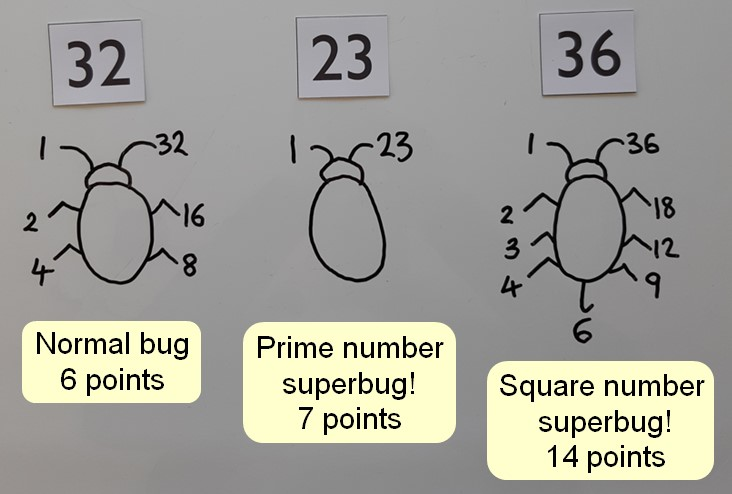## Superbug smash!

A game for 2 or more players

This game provides practice of finding all the factors of a number and recognising prime numbers and square numbers. It is played in the same way as Bug battles! with bonus points being awarded for the superbugs – the prime numbers and square numbers.

### You will need:

• Pen and paper:
• Number cards (choose numbers you want to find factors of, including at least the first ten square numbers and prime numbers)
• A multiplication square can be used for support as needed
• ### How to play

Agree how many rounds will be played.

Players each draw a card and then make a factor bug to record the factor pairs.

Each person scores points equal to the number of factors their bug has.

Players score 4 bonus points for a prime number and 2 bonus points for a square number.

The winner is the person with the most points after the agreed number of rounds.

### Variations

• Play using an appropriate dice instead of number cards
• Turn over cards equal to the number of players, then each player in turn chooses one of the cards. A different player should choose first each round.
• Find out more about factors

Find out more about prime numbers

Find out more about square numbers